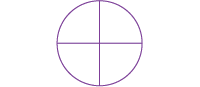Home > Fractions > Good teaching > Fraction sense > Visualisation

# Visualisation

A commonly used representation for fractions is the area diagram, such as circles or squares.Area diagram showing quarters.

Care must be taken to ensure students understand what the 'whole' actually is, and that includes understanding what area means.

What fraction of a circle is represented by this shape?Diagram to prompt visualisation of the whole.

Students with insufficient fraction sense are not able to visualise the whole, or how many equal parts are involved. Therefore they would be unable recognise the above shape as representing three-quarters of the area of a circle.

If students are always shown representations of fractions where all the dividing lines are drawn and all the parts are visible, they never have to visualise where those lines would be.

Teachers can incorporate opportunities for building fraction visualisation skills in activities that require students to make their own representations of fractions.

## Fraction wheel

Students can use a fraction wheel made from two paper plates to model fractions and build visualising skills.

## Cake fractions

Students use a digital learning object to predict the written fraction that best describes part of a circle. Students then reconstruct both the symbolic fraction and the area model to check the prediction.